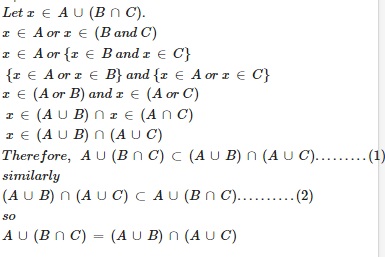# Maths Sets - Online Test

Q1. If A = { 1,2,3 } , and B = { 1,3,5,7 } , then A∪B=
Explaination / Solution: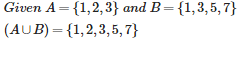Q2. If A = { 1,2,3,4 } , B = { 4,5,6,7 } ,A∩B=
Explaination / Solution: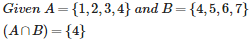Q3. Let A = { 1,2,3,4 } then total number of subsets of A are given by
Explaination / Solution:

Total no of subsets=2n=24=16

Here n is the Cardinal no of the Given Set

Q4. If A = { 1,2,3,4 } and B = { 4,5,6,7 } then A−B =
Explaination / Solution:

Given A={1,2,3,4} and B={4,5,6,7}

A-B set will contain those elements which are present in A but not in B

we can see

Now

so

Q5. If n (A ) =3 and n ( B ) = 6 and A⊆ B , then n(A∪B)=?
Explaination / Solution: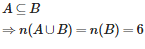Q6. If n (A ) =3 and n ( B ) = 6 and A⊆ B , then n(A∩B) is equal to
Explaination / Solution:Q7. Let A and B be subsets of a set X , Then which of the following is correct
Explaination / Solution: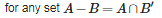Q8. If A , B and C are any three sets , then A – ( B ∪ C ) is equal to
Explaination / Solution: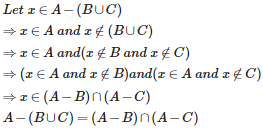Q9. If A, B and C are any three sets , then A – (B∩∩C ) is equal to
Explaination / Solution: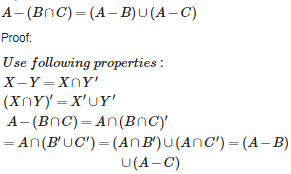Q10. If A, B and C are any three sets , then A ∩ ( B ∪C ) is equal to
Explaination / Solution: